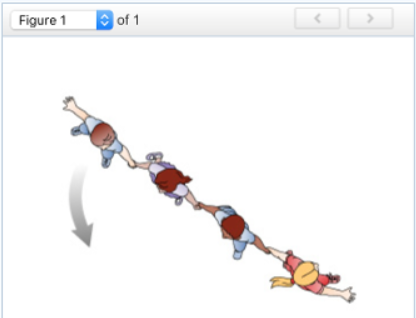# Problem: Four children are playing in a field. The children form a line, holding hands. The player at the front of the line starts to spin around faster and faster, causing the others to run around her in a circle, as shown in (Figure 1).Part 1While the line of children is rotating, which of the following statements are correct? Check all that apply.a) The player at the front of the line has the smallest angular acceleration.b) The player at the front of the line has the smallest linear velocity.c) All the children have the same angular acceleration.d) All the children have the same linear velocity.Part 2Now consider the children's linear accelerations. Which of the following statements are correct? Check all that apply.a) The last child in the line has the greatest tangential acceleration.b) The last child in the line has the greatest radial acceleration.c) All the children have the same tangential acceleration.d) All the children have the same radial acceleration.

###### FREE Expert Solution

Angular velocity,

$\overline{){\mathbf{\omega }}{\mathbf{=}}\frac{\mathbf{∆}\mathbf{\theta }}{\mathbf{∆}\mathbf{t}}}$

Angular acceleration,

$\overline{){\mathbit{\alpha }}{\mathbf{=}}\frac{\mathbf{∆}\mathbit{\omega }}{\mathbf{∆}\mathbf{t}}}$

Δθ is the same for all, while Δθ•r is different for each.

92% (436 ratings)###### Problem Details

Four children are playing in a field. The children form a line, holding hands. The player at the front of the line starts to spin around faster and faster, causing the others to run around her in a circle, as shown in (Figure 1).Part 1

While the line of children is rotating, which of the following statements are correct? Check all that apply.

a) The player at the front of the line has the smallest angular acceleration.

b) The player at the front of the line has the smallest linear velocity.

c) All the children have the same angular acceleration.

d) All the children have the same linear velocity.

Part 2

Now consider the children's linear accelerations. Which of the following statements are correct? Check all that apply.

a) The last child in the line has the greatest tangential acceleration.

b) The last child in the line has the greatest radial acceleration.

c) All the children have the same tangential acceleration.

d) All the children have the same radial acceleration.